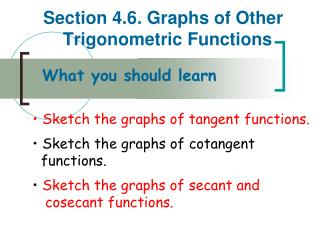Download PresentationSection 4.6. Graphs of Other Trigonometric Functions

Section 4.6. Graphs of Other Trigonometric Functions - PowerPoint PPT Presentation

Section 4.6. Graphs of Other Trigonometric Functions. What you should learn Sketch the graphs of tangent functions. • Sketch the graphs of cotangent functions. • Sketch the graphs of secant and cosecant functions. Problem of the Day.I am the owner, or an agent authorized to act on behalf of the owner, of the copyrighted work described.
Download PresentationSection 4.6. Graphs of Other Trigonometric Functions

Download Policy: Content on the Website is provided to you AS IS for your information and personal use and may not be sold / licensed / shared on other websites without getting consent from its author.While downloading, if for some reason you are not able to download a presentation, the publisher may have deleted the file from their server.

- - - - - - - - - - - - - - - - - - - - - - - - - - E N D - - - - - - - - - - - - - - - - - - - - - - - - - -
Presentation Transcript
1. Section 4.6. Graphs of Other Trigonometric Functions • What you should learn • Sketch the graphs of tangent functions. • • Sketch the graphs of cotangent • functions. • • Sketch the graphs of secant and • cosecant functions.

2. Problem of the Day

3. Graph of the Tangent Function y = tan x Recall that the tangent function is odd, thus tan (-x) = -tan x. Therefore, the graph of y = tan x is symmetric with respect to the origin.

4. Transforming a Tangent Functiony = a tan (bx - c) • Two consecutive vertical asymptotes can be found by solving the equations bx – c = - π/2 and bx – c = π/2 • Theperiodof the function y = a tan (bx - c) is the distance between two consecutive vertical asymptotes. • The midpoint between two vertical asymptotes is an x-intercept of the graph. • The amplitudeof the tangent function is undefined.

5. Example 1. Sketching the Graph of a Tangent Function

6. Example 2.Sketching the Graph of a Tangent Function

7. Problem of the Day

8. Graph of the Cotangent Function • The graph of the cotangent function is similar to the graph of the tangent function. • It has a period of π. • Since , The cotangent function has vertical asymptotes when sin x is zero, which occurs at x = nπ, where n is an integer

9. Compare and Contrast Tangent and Cotangent

10. Graph of the Cotangent Function • Two consecutive vertical asymptotes can be found by solving the equations bx – c = 0 and bx – c = π

11. Example 3.Sketching the Graph of a Cotangent Function

12. Graphs of the Reciprocal Functions

13. Graph of the Cosecant Function • Sketch the graph of:

14. Graph of the Secant Function • Sketch the graph of: y = sec 2x

15. Assignment 4-6 4.6 Exercises p. 339 1-6 all p. 368 141-144 all